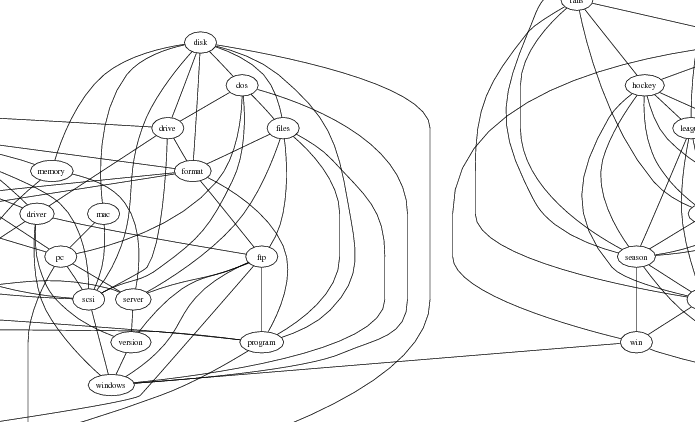# L1precision

Mark Schmidt (2006)

This is a Matlab function implementing maximum a posteriori (MAP) estimation of the precision matrix in a generative Gaussian Graphical Model (GGM), where a Laplace prior (ie. L1-regularizer of the negative log-likelihood) has been placed on the values of the precision matrix (also known as the `Graphical Lasso'). Formally, for a given empirical covariance matrix S and a regularizer strength v, we seek to minimize the following expression in terms of the matrix X:

log |X| - < S,X > - v||X||_1

In the above |X| denotes the determinant of X, < S,X > is the matrix inner product of S and X, and ||X||_1 is the sum of the absolute values of the elements of X (NOT the matrix L1-norm). To form a proper Precision/Covariance, this optimization must be performed subject to symmetry of the matrix and a positive-definite constraint.

This constrained optimization is solved using the method of (Banerjee, El Ghaoui, d'Aspremont, and Natsoulis. "Convex optimization tehcniques for fitting sparse Gaussian graphical models", ICML 2006), where Block Coordinate Descent is applied to the convex dual, and each column of the matrix is optimized in turn as a bound-constrained Quadratic Program.

There are 2 avialable options for solving the Quadratic Programs: If the Quadratic Programming in C (QPC) mex files are available, then the qpas mex file from this software will be used. Otherwise, the method formulates the block update as a Lasso optimization problem and solves the Lasso optimization using the `Shooting' algorithm (Knight and Fu, "Penalized regressions: the bridge vs the lasso", JCGS 98), as in the Graphical Lasso method.

This code was used in the paper Modeling changing dependency structure in multivariate time series by Xiang Xuan and Kevin Murphy (ICML, 2007).

### Usage

The function has the following interface:
X = L1precisionBCD(S,v);

### Example

Running 'example_L1precision' will apply this function to a word co-occurrence data set with v set to 1. Below, a portion of the resulting graph is shown:The full graph can be viewed as a PDF here.

The complete set of .m files are available here. The QPC software is available here.

### Citations

If you use L1precision in a publication, please cite the work using the following information:
• M. Schmidt. L1precision: Matlab code for MAP estimation of Gaussian Graphical Model Precision with L1-regularizer. http://www.cs.ubc.ca/~schmidtm/Software/L1precision.html, 2006.

### Other Optimization Strategies

Since estimating Gaussian graphical models with L1-regularization was originally proposed, a variety of alternatives to block coordinate descent have been proposed for solving the optimization problem. In some cases these can be much more efficient. A variety of alternative optimization strategies are available via the L1General and minConf codes (for solving the primal and dual problems, respectively). The Examples of using L1General page shows explicitly how to use the L1General code to solve this (graphical LASSO) optimization problems.

### Blockwise-Sparsity

As an alternative to L1-regularization, group L1-regularization can be used to encourage sparsity in the blocks of the precision matrix between pre-specified 'types' of variables. This approach was originally proposed in (Duchi, Gould, and Koller. "Projected Subgradient Methods for Learning Sparse Gaussians", UAI 2008). This optimization problem can be solved using the SPG or PQN methods present in the minConf package. The Examples of using minConf_PQN shows how to do this explicitly.

Mark Schmidt > Software > L1precision